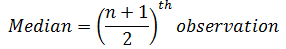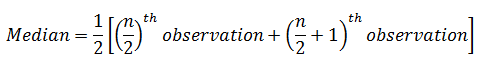# Median Of Numbers

What is the Median of Numbers?

One of the measures of central tendency is median. Median of numbers is the middlemost value of the given set of numbers. It separates the higher half and the lower half of a given data sample. At least half of the observations are smaller than or equal to median and at least half of the observations are greater than or equal to the median.

## How to find the median of numbers?

In order to find the median of numbers, we first need to arrange the given numbers either in ascending order or in descending order of their numerical value. It is now easy to find the median after arranging the given numbers.

## Median of Odd Numbers

If there are odd number of observations in a data set, then the median can be calculated as below:

Step 1: Arrange the data either in ascending or in descending order.

Step 2: If the number of observations (say n) is odd, then the middlemost observation is the median of the given data.## Median of Even Numbers

If there are even number of observations in a data set, then the median can be calculated as below:

Step 1: Arrange the data either in ascending or in descending order.

Step 2: If the number of observations (say n) are even, then identify (n/2)th and [(n/2) + 1]th observations.

Step 3: The average of the above two observations (which are identified in step 2) is the median of the given data.Difference Between Mean Median And Mode

Median Data

Statistics For Class 10

Important Questions Class 9 Maths Chapter 14 Statistics

### Median of Two Numbers

If there are only two numbers in a data set, then their median will be the same as their mean (or arithmetic mean or average). For example, the mean and median of 6 and 14 is equal to 10.

### Median of Three Numbers

If there are three numbers in a data set, then first arrange them either in ascending order or in descending order. Now the middle-most number is the median of the given data set. For example, 17, 21, and 11 are three numbers of a data set. Now, the descending order of the numbers is 21, 17, 11. Hence,17 is the median.

### Median of a list of Numbers

The median is the value which lies at the midpoint of the data set but it is not the midpoint of the values. It doesn’t mean anything for two numbers only. Consider the below illustration to understand in a better way.

Let us consider 12 and 6.

Mean = Median = (12 + 6)/2 = 9

Adding 2 more values to the above data set.

Now, 12, 6, 7, 5

Ascending order: 5, 6, 7, 12

Median = (6 + 7)/2 = 6.5

Mean = (12 + 6 + 7 + 5)/4 = 30/4 = 7.5

Similarly, if we add one more value to the data set, the median will shift.

Example 1:

The marks scored by 12 students of a class are given by:

40, 50, 60, 55, 70, 45, 60, 80, 65, 50, 82, 58

Find the median marks of the given students.

Solution:

Given marks are:

40, 50, 60, 55, 70, 45, 60, 80, 65, 50, 82, 58

Ascending order:

40, 45, 50, 50, 55, 58, 60, 60, 65, 70, 80, 82

12 is an even number, i.e. n = 12 and n/2 = 12/2 = 6.

6th observation is 58.

7th observation is 60.

Median = (58 + 60)/2 = 118/2 = 59

Example 2:

Given below are the ages (in years) of 15 employees in the HR department of a company.

24, 49, 35, 27, 36, 40, 30, 25, 32, 26, 34, 40, 42, 28, 32

Find the median age of the employees in the HR department.

Solution:

Given data:

24, 49, 35, 27, 36, 40, 30, 25, 32, 26, 34, 40, 42, 28, 32

Ascending order:

24, 25, 26, 27, 28, 30, 32, 32, 34, 35, 36, 40, 40, 42, 49

15 is an odd number, i.e. n = 15 and (n+1)/2 = (15 + 1)/2 = 8

8th observation = 32

Hence, the median age = 32 years

Quiz on Median of numbers